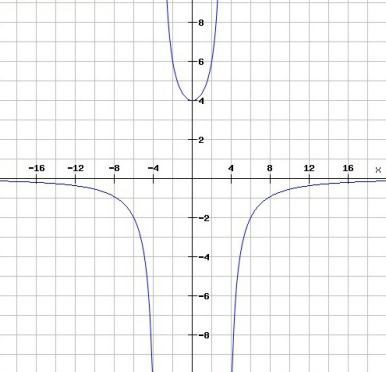# Free SAT Test Questions

Question 1 of 1
ID: SAT-HA-9
Section: Math - Heart of Algebra
Topic: Graphs
Difficulty level: Hard

(Practice Mode: Single selected Question » Back to Overview)Which of the following best describes the relation between $x$ and $y$ in the above graph? (Without calculator)

A$y=\frac{32}{x^2}$
B$y=\frac{48}{12+x^2}$
C$y=\frac{48}{12-x^2}$
D$y=\frac{48}{x^2-12}$

#### Finding the Right Time to Take the SAT

Read on the optimal timings to sit for the SAT that will give you a competitive edge. With the SAT only being offered a few times every year, it is critical to find the right time to take your exam.